TitleIntermediate Algebra
Answer/Discussion to Practice Problems
Tutorial 16: Equations of LinesAnswer/Discussion to 1a Slope 2/3; y-intercept (0, -2)

 Since we have the slope and the y-intercept, then we can go right into plugging in 2/3  for m (slope) and -2 for b (y-intercept) into the slope/intercept form and we will have our equation.*Slope/intercept form of a line

 y = 2/3 x - 2 is the equation of the line that has a slope of 2/3 and y-intercept of (0, -2). (return to problem 1a)Answer/Discussion to 2a 3x + 5y = 10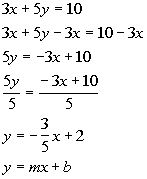*Inverse of add 3x is sub. 3x   *Inverse of mult. by 5 is div. by 5   *Slope/intercept form of the line

 Looking at this equation and lining it up with the slope/intercept form, what do you get for the slope and y-intercept? I got m = -3/5 and y-intercept is 2.The slope is -3/5.  Starting on the y-intercept (2, 0), we will go down 3 and run right 5.Step 5: Draw the graph.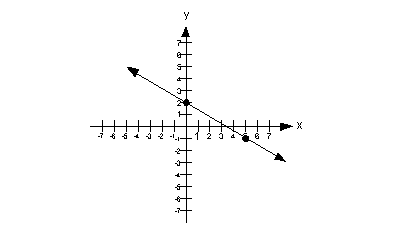(return to problem 2a)Answer/Discussion to 3a  Passes through (-3, 2) and has a slope of -1/2.

 What are the two things we need to write an equation of a line???? If you said any point on the line and the slope, you are correct. Looks like we have all the information we need.  We are ready to put our equation together.  Since we don't have the y-intercept, we will have to use the point/slope form since that is set up for ANY point (not just the y-int.).*Point/slope form of the line

 Next, we want to write it in the slope/intercept form, which basically means we need to solve for y.*Dist. the -1/2 through (  )   *Inverse of sub. 2 is add 2 *LCD is 2 *Mult. 2/1 by 2/2 to get 4/2 *Slope/intercept form of the line

 The equation of the line that passes through (-3, 2) and has a slope of -1/2 is y = -1/2 x + 1/2.  (return to problem 3a)Answer/Discussion to 4a  (0, 0) and (5, 10)

 What are the two things we need to write an equation of a line???? If you said any point on the line and the slope, you are correct. We have more than enough points. However, what about the slope?  Does this mean we can't work out the problem?  You are not going to get off that easily.  We do have a way of finding the slope. Tutorial 15: The Slope of a Line shows us how we can get the slope given two points. Let's find that slope:*Slope formula *Plug in values  *Simplify

 OK, now we have our slope, which is 2.  Now we want to put the slope and one point into the point/slope equation.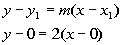*Point/slope form of the line

 The directions said to write it using function notation. This means we want to solve it for y (get it in the slope/int form) and then put it the function notation.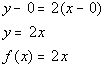*Dist. 2 through the (  ) *Slope/intercept form of the line  *Function notation

 The line that passes through (0, 0) and (5, 10) in function notation is f(x) = 2x. (return to problem 4a)Answer/Discussion to 5a  Slope 0; passes through (1, 2)

 Recall from Tutorial 14: Graphing Equations that a horizontal line is of the form y = c and from Tutorial 15: The Slope of the Line that a horizontal line has a slope of 0. It is one of our 'special' types of lines that does not have both x and y showing in the equation.  So, we will not use the point/slope form, but go right into setting up the equation y = c. Since it passes through (1, 2), and a horizontal line is in the form y = c, where the y value is ALWAYS equal to the same value throughout, this means our equation would have to be y = 2. Note that 2 is the y value of the ordered pair given. (return to problem 5a)Answer/Discussion to 5b  Vertical; passes through (-1, -2)

 Recall from Tutorial 14: Graphing Equations and Tutorial 15: The Slope of the Line that the 'special' type of line that has an undefined slope is a vertical line and a vertical line is of the form x = c.  So, we will not use the point/slope form, but go right into setting up the equation x = c. Since it passes through (-1, -2), and a vertical line is in the form x = c, where the x value is ALWAYS equal to the same value throughout, this means our equation would have to be x = -1. Note that -1 is the x value of the ordered pair given. (return to problem 5b)Answer/Discussion to 6a  Passes through (2, 3) and parallel to 5x + 2y = 4

 What are the two things we need to write an equation of a line???? If you said any point on the line and the slope, you are correct. We have our point. However, what about the slope?  We need to do a little digging to get it. Recall that Tutorial 15: The Slope of a Line tells us that parallel lines have the same slope.  So, if we know the slope of the line parallel to our line, we have it made. Find the slope of the parallel line: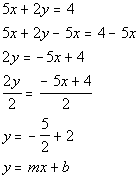*Inverse of add 5x is sub. 5x   *Inverse of mult. by 2 is div. by 2   *Slope/intercept form of the line

 Now, keep in mind that this is not the equation of our line but of the line parallel to our line.  We needed to write it this way so we could get the slope.  And it looks like the slope is -5/2. And since our line is parallel to a line that has a slope of -5/2, our line also has a slope of -5/2.   OK, now we have our slope, which is -5/2.  Now we want to put the slope and one point into the point/slope equation.*Point/slope form of the line

 The directions said to write it using function notation. This means we want to solve it for y (get it in the slope/int form) and then put it in function notation.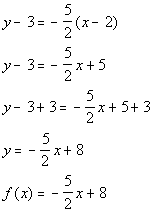*Dist. -5/2 through (   )     *Inverse of sub. 3 is add 3 *Slope/intercept form of the line *Function notation

 The line that passes through (2, 3) and is parallel to 5x + 2y = 4 is f(x) = -5/2 x + 8. (return to problem 6a)Answer/Discussion to 6b  Passes through (-1, 0) and perpendicular to 3x - y = 2

 What are the two things we need to write an equation of a line???? If you said any point on the line and the slope, you are correct. We have our point. However, what about the slope?  We need to do a little work in that department. Recall that  Tutorial 15: The Slope of a Line tells us that the slopes of perpendicular lines are negative reciprocals of each other.   So, if we know the slope of the line perpendicular to our line, we have it made. Find the slope of the perpendicular line: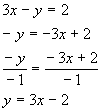*Subtract 3x from both sides *Inverse of mult. by -1 is div. by -1 *Slope/intercept form of the line

 In this form, we can tell that the slope of this line is 3.  Since our line is perpendicular to this, we need to take the negative reciprocal of 3 to get our slope.  What did you come up with?  I came up with -1/3 for the slope of our line.  Now we can go on to the equation of our line: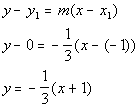*Point/slope form of the line

 The directions said to write it using function notation. This means we want to solve it for y (get it in the slope/int form) and then put it in function notation.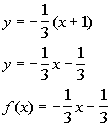*Dist. -1/3 through the (   ) *Slope/intercept form of the line *Function notation

 The line that passes through (-1, 0) and is perpendicular to 3x - y = 2  is f(x) = -1/3 x - 1/3. (return to problem 6b)

Last revised on July 5, 2011 by Kim Seward.
All contents copyright (C) 2001 - 2011, WTAMU and Kim Seward. All rights reserved.